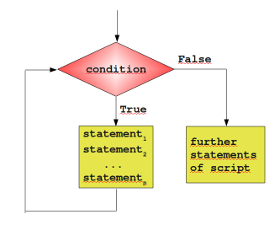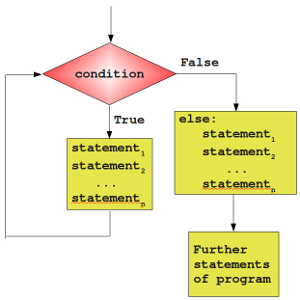## Loops

### General Structure of a LoopMany algorithms make it necessary for a programming language to have a construct which makes it possible to carry out a sequence of statements repeatedly. The code within the loop, i.e. the code carried out repeatedly is called the body of the loop.
Python supplies two different kinds of loops: the while loop and the for loop.

Most loops contain a counter or more generally variables, which change their values in the course of calculation. These variables have to be initialized before the loop is started. The counter or other variables, which can be altered in the body of the loop, are contained in the condition. Before the body of the loop is executed, the condition is evaluated. If it evaluates to True, the body gets executed. After the body is finished, the condition will be evaluated again. The body of the loop will be executed as long as the condition yields True.

### A Simple Example with a While Loop

It's best to illustrate the operating principle of a loop with a simple example. The following small script calculates the sum of the numbers from 1 to 100. We will later introduce a more elegant way to do it.

#!/usr/bin/env python

n = 100

sum = 0
i = 1
while i <= n:
sum = sum + i
i = i + 1

print "Sum of 1 until %d: %d" % (n,sum)


Before we go on with the while loop, we want to introduce some fundamental things on standard input and standard output. Normally, the keyboard serves as the standard input. The standard output is usually the terminal or console where the script had been started, which prints the output. A script is supposed to send its error messages to the standard error.
Python has these three channels as well:
• standard input
• standard output
• standard error
They are contained in the module sys. Their names are:
• sys.stdin
• sys.stdout
• sys.stderror
.

The following script shows how to read with a while loop character by character from standard input (keyboard).

import sys

text = ""
while 1:
text = text + c
if c == '\n':
break

print "Input: %s" % text

Though it's possible to read input like this, usually the function raw_input() is used.
>>> name = raw_input("What's your name?\n")
Tux
>>> print name
Tux
>>>


### The else PartSimilar to the if statement, the while loop of Python has also an optional else part. This is an unfamiliar construct for many programmers of traditional programming languages.
The statements in the else part are executed, when the condition is not fulfilled anymore. Some might ask themselves now, where the possible benefit of this extra branch is. If the statements of the additional else part were placed right after the while loop without an else, they would have been executed anyway, wouldn't they. We need to understand a new language construct, i.e. the break statement, to obtain a benefit from the additional else branch of the while loop.
The general syntax of a while loop looks like this:

 while condition: statement_1 ... statement_n else: statement_1 ... statement_n 

### Premature Termination of a while LoopSo far, a while loop only ends, if the condition in the loop head is fulfilled. With the help of a break statement a while loop can be left prematurely, i.e. as soon as the control flow of the program comes to a break inside of a while loop (or other loops) the loop will be immediately left. "break" shouldn't be confused with the continue statement. "continue" stops the current iteration of the loop and starts the next iteration by checking the condition.
Now comes the crucial point: If a loop is left by break, the else part is not executed.

This behaviour will be illustrated in the following example, a little guessing number game. A human player has to guess a number between a range of 1 to n. The player inputs his guess. The program informs the player, if this number is larger, smaller or equal to the secret number, i.e. the number which the program has randomly created. If the player wants to gives up, he or she can input a 0 or a negative number.
Hint: The program needs to create a random number. Therefore it's necessary to include the module "random".

import random
n = 20
to_be_guessed = int(n * random.random()) + 1
guess = 0
while guess != to_be_guessed:
guess = input("New number: ")
if guess > 0:
if guess > to_be_guessed:
print "Number too large"
else:
print "Number too small"
else:
print "Sorry that you're giving up!"
break
else: